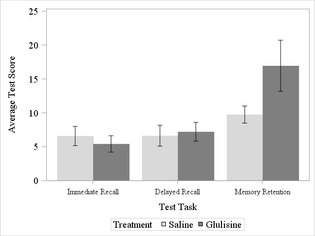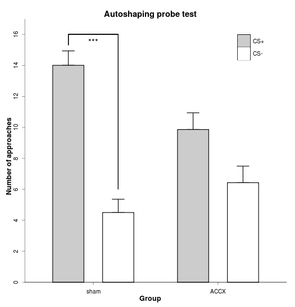## Adding significance star on VBAR in SGPLOT

Hello,

I'm attempting to avoid my instinct to switch to R when doing something graphical. Is there a way to add a significance star, p-value and/or connector above my clustered vertical bars to show a statistical difference between groups?

Current code:

```proc sgplot data=cog_long;
styleattrs datacolors=(%RGB(217,217,217) %RGB(127,127,127));
vbar testnum / response=score stat=mean limitstat=stderr
limitattrs=(color=black) group=trt groupdisplay=cluster
nooutline;
xaxis valueattrs=(size=12pt family="Times New Roman")
labelattrs  =(size=14pt family="Times New Roman");
yaxis min=0 max=25 valueattrs=(size=14pt family="Times New Roman")
labelattrs=(size=14pt family="Times New Roman");
keylegend / titleattrs=(size=14pt family="Times New Roman")
valueattrs=(size=14pt family="Times New Roman");
title;
run;```

Current graph:Note: significance star/connector would go above Memory Retention

The desired graph would have connecting line with ** above the Memory Retention tests similar to this graph produced in R:Ksharp
Super User

## Re: Adding significance star on VBAR in SGPLOT

You could use

BARPARM  statement + SCATTER statement (plot a big Star symbol)

Discussion stats